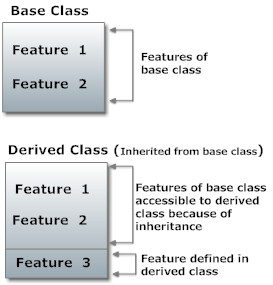# Python Inheritance

Date:

Inheritance is a powerful feature in object-oriented programming. It refers to defining a new class with little or no modification to an existing class. The new class is called the derived (or child) class and the one from which it inherits is called the base (or parent) class. A derived class inherits features from the base class, adding new features to it. This results into re-usability of code.## Python Inheritance Syntax

```class DerivedClass(BaseClass):
body_of_derived_class```

## Example of Inheritance in Python

To demonstrate the use of inheritance, let us take an example. A polygon is a closed figure with 3 or more sides. Say, we have a class called `Polygon` defined as follows.

```class Polygon:
def __init__(self, no_of_sides):
self.n = no_of_sides
self.sides = [0 for i in range(no_of_sides)]

def inputSides(self):
self.sides = [float(input("Enter side "+str(i+1)+" : ")) for i in range(self.n)]

def dispSides(self):
for i in range(self.n):
print("Side",i+1,"is",self.sides[i])```

This class has data attributes to store the number of sides, n, and magnitude of each side as a list, sides. Method `inputSides()` takes in the magnitude of each side and similarly, `dispSides()` will display these properly.

A triangle is a polygon with 3 sides. So, we can create a class called `Triangle` which inherits from`Polygon`. This makes all the attributes available in class `Polygon` readily available in `Triangle`. We don’t need to define them again (code re-usability). `Triangle` is defined as follows.

```class Triangle(Polygon):
def __init__(self):
Polygon.__init__(self,3)

def findArea(self):
a, b, c = self.sides
# calculate the semi-perimeter
s = (a + b + c) / 2
area = (s*(s-a)*(s-b)*(s-c)) ** 0.5
print('The area of the triangle is %0.2f' %area)```

However, class `Triangle` has a new method `findArea()` to find and print the area of the triangle. Here is a sample run.

```>>> t = Triangle()

>>> t.inputSides()
Enter side 1 : 3
Enter side 2 : 5
Enter side 3 : 4

>>> t.dispSides()
Side 1 is 3.0
Side 2 is 5.0
Side 3 is 4.0

>>> t.findArea()
The area of the triangle is 6.00```

We can see that, even though we did not define methods like `inputSides()` or `dispSides()` for class `Triangle`, we were able to use them. If an attribute is not found in the class, search continues to the base class. This repeats recursively, if the base class is itself derived from other classes.

## Method Overriding in Python

In the above example, notice that `__init__()` method was defined in both classes, `Triangle` as well`Polygon`. When this happens, the method in the derived class overrides that in the base class. This is to say, `__init__()` in `Triangle` gets preference over the same in `Polygon`. Generally when overriding a base method, we tend to extend the definition rather than simply replace it. The same is being done by calling the method in base class from the one in derived class (calling `Polygon.__init__()` from`__init__()` in `Triangle`). A better option would be to use the built-in function `super()`. So,`super().__init(3)` is equivalent to `Polygon.__init__(self,3)` and is preferred. You can learn more about the super() function in Python.

Two built-in functions `isinstance()` and `issubclass()` are used to check inheritances. Function`isinstance()` returns `True` if the object is an instance of the class or other classes derived from it. Each and every class in Python inherits from the base class `object`.

```>>> isinstance(t,Triangle)
True

>>> isinstance(t,Polygon)
True

>>> isinstance(t,int)
False

>>> isinstance(t,object)
True```

Similarly, `issubclass()` is used to check for class inheritance.

```>>> issubclass(Polygon,Triangle)
False

>>> issubclass(Triangle,Polygon)
True

>>> issubclass(bool,int)
True
```

Share post:

Popular

### Tango Live: Free Guide to Exclusive Features and Benefits

Introduction Welcome to our comprehensive guide on Tango Live, the...

### How to Setup SailPoint and Manage IdentityIQ with Compass

Introduction to SailPoint Technology and SailPoint Compass: A Comprehensive...